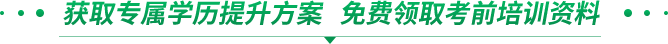# 成人高考专升本高等数学（二）模拟题1、在空间直角坐标系中，方程2+3y2+3x2=1表示的曲面是( )．

A．球面B．柱面C．锥面D．椭球面

2.设函数f（x)=2sinx，则f′(x)等于( )．

A．2sinx B．2cosx C．-2sinx D．-2cosx

3.设y=lnx，则y″等于( )．

A．1/x B．1/x2C．-1/xD．-1/x2

4.方程z=x2+y2表示的二次曲面是( )．

A．球面B．柱面C．圆锥面D．抛物面

5.设y=2x3，则dy=( )．

A．2x2dx B．6x2dx C．3x2dxD．x2dx

6.微分方程(y′)2=x的阶数为( )．

A．1 B．2 C．3 D．4

7.过点(1，0，0)，(0，1，0)，(0，0，1)的平面方程为( )．

A．x+y+z=1 B．2x+y+z=1 C．x+2y+z=1 D．x+y+2z=1

8.曲线y=x3+1在点(1，2)处的切线的斜率为( )．

A．1 B．2 C．3 D．4

9.设函数f(x)在[0，b]连续，在(a，b)可导，f′(x)>0．若f(a)·f(b)<0，则y=f(x)在(a，b)( )．

A．不存在零点

B．存在唯一零点

C．存在极大值点

D．存在极小值点

10.设Y=e-3x，则dy等于( )．

A．e-3xdx B．-e-3xdx C．-3e-3xdx D．3e-3xdx

11、将ex展开为x的幂级数，则展开式中含x3项的系数为_____.

12、设y=3+cosx，则y′_____.

13、设y=fx)可导，点a0=2为fx)的极小值点，且f2)=3，则曲线y=f(x)在点(2，3)处的切线方程为______．

14、设函数z=ln(x+y2)，则全微分dz=_______.

15、 过M设y=f(x)在点x=0处可导，且x=0为f(x)的极值点，则f′(0)=_____.

16、 (1，-l，2)且垂直于平面2x-y+3z-1=0的直线方程为_____.

17、 微分方程y′=0的通解为_____.

18、 过M(1，-l，2)且垂直于平面2x-y+3z-1=0的直线方程为_____.

19、 设y=2x2+ax+3在点x=1取得极小值，则a=_____.

20、 微分方程xyy′=1-x2的通解是_____.

21、 求函数y=x-lnx的单调区间，并求该曲线在点(1，1)处的切线l的方程．

22、设z=z(x，Y)是由方程z+y+z=ez所确定的隐函数，求dz．

23、求函数f(x)=x3-3x+1的单调区间和极值．

24、设l是曲线y=x2+3在点(1，4)处的切线，求由该曲线，切线l及Y轴围成的平面图形的面积S．

25、求微分方程y"-y′-2y=3ex的通解．

26、设F(x)为f(x)的一个原函数，且f(x)=xlnx，求F(x).

27、设F(x)为f(x)的一个原函数，且f(x)=xlnx，求F(x).

28、设y=x+sinx，求y′>25、求微分方程y"-y′-2y=3ex的通解．×